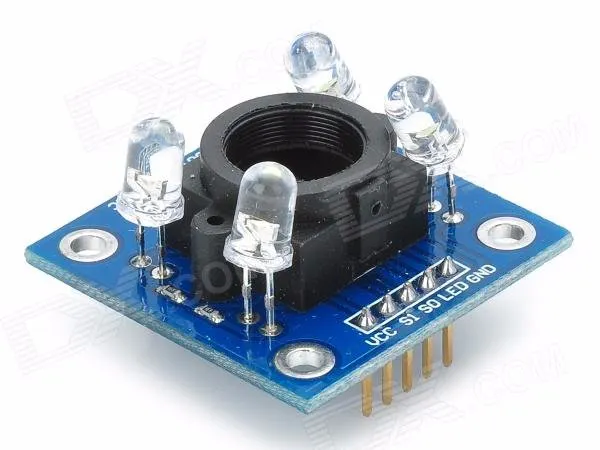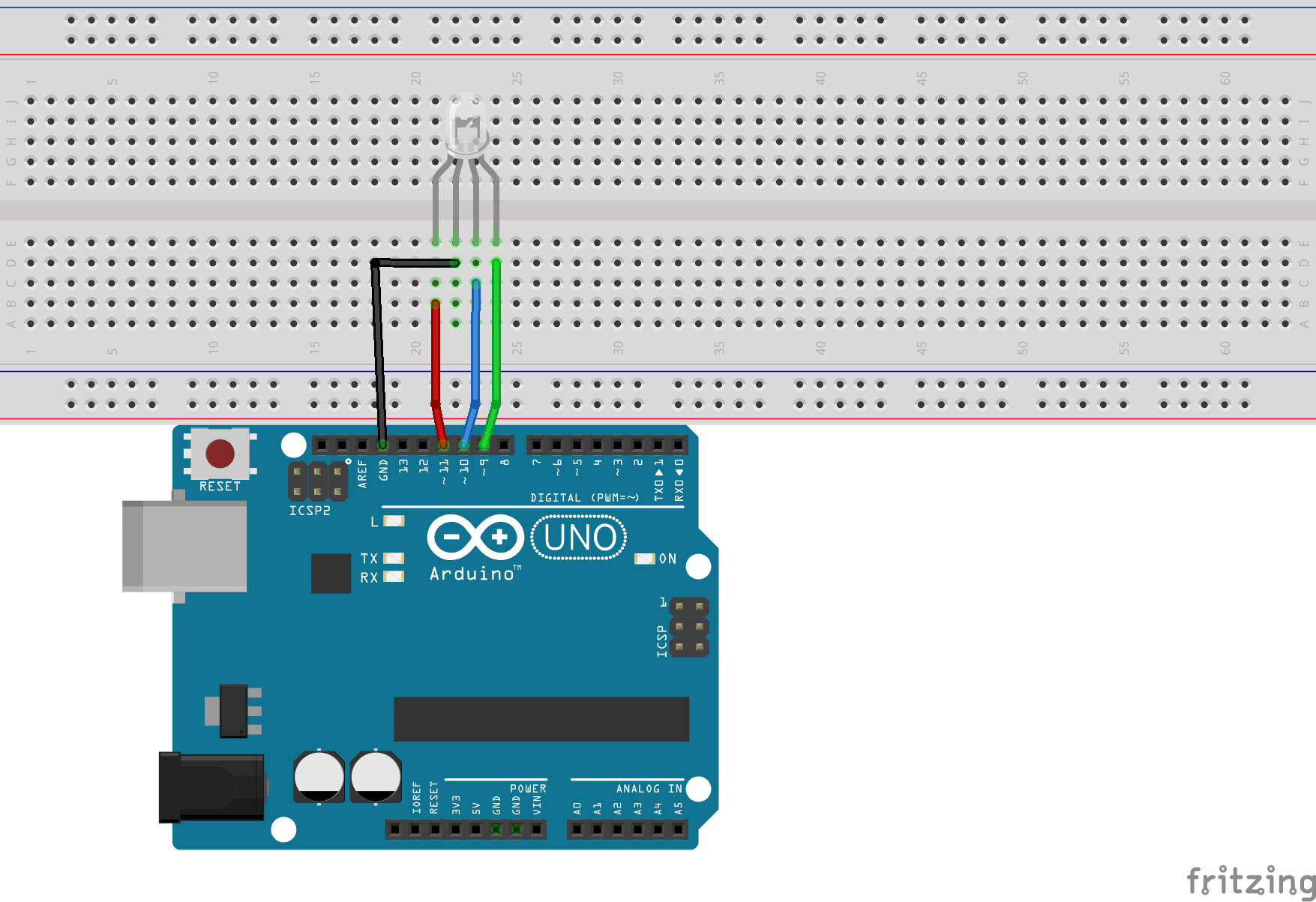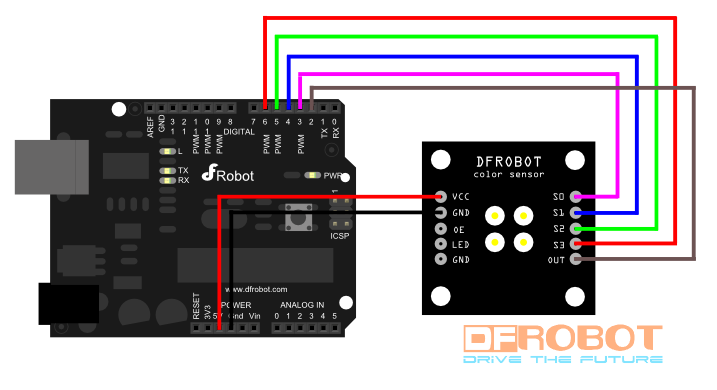# Arduino Chameleon Using TCS3200 © GPL3+

In this project I will guide you on how to make your own Arduino Chameleon using TCS3200 and Arduino Uno. ​

• 2,741 views
• 3 respects

## Components and supplies

In this project I will guide you on how to make your own Arduino Chameleon using TCS3200 and Arduino Uno. For more info and Arduino and Raspberry Pi related projects go to my website: https://www.neuralnetworks26.com/

### Parts Needed

• TCS3200 x 1
• Arduino Uno x 1
• RGB LED x 1
• A lot of jumper wires

• Arduino IDE

### Steps to be Followed

• Please refer to the following images for all the wire connections.

• Now just upload the following code onto your Arduino and it's done.
``````/*
* Created By Manan Thareja
* Color sensor using TCS3200
*/
#define S0 3
#define S1 4
#define S2 5
#define S3 6
#define sensorOut 2
int green = 9;
int blue = 10;
int red  = 11;
int redfrequency = 0;
int greenfrequency = 0;
int bluefrequency = 0;
void setup() {
pinMode(S0, OUTPUT);
pinMode(S1, OUTPUT);
pinMode(S2, OUTPUT);
pinMode(S3, OUTPUT);
pinMode(sensorOut, INPUT);
pinMode(green, OUTPUT);
pinMode(blue, OUTPUT);
pinMode(red, OUTPUT);
// Setting frequency-scaling to 20%
digitalWrite(S0,HIGH);
digitalWrite(S1,LOW);
Serial.begin(9600);
}
void loop() {
// Setting red filtered photodiodes to be read
digitalWrite(S2,LOW);
digitalWrite(S3,LOW);
redfrequency = pulseIn(sensorOut, LOW);
//Remaping the value of the frequency to the RGB Model of 0 to 255
redfrequency = map(redfrequency, 25,72,255,0);
if (redfrequency > 255)
{
redfrequency = 255;
}
else if (redfrequency < 0)
{
redfrequency = 0;
}
// Printing the value on the Serial monitor
Serial.print("R ");//printing name
Serial.print(redfrequency);//printing RED color frequency
analogWrite(red, redfrequency);
delay(100);
// Setting Green filtered photodiodes to be read
digitalWrite(S2,HIGH);
digitalWrite(S3,HIGH);
greenfrequency = pulseIn(sensorOut, LOW);
//Remaping the value of the frequency to the RGB Model of 0 to 255
greenfrequency = map(greenfrequency, 30,90,255,0);
if (greenfrequency > 255)
{
greenfrequency = 255;
}
else if (greenfrequency < 0)
{
greenfrequency = 0;
}
// Printing the value on the Serial monitor
Serial.print(" G ");//printing name
Serial.print(greenfrequency);//printing GREEN color frequency
analogWrite(green, greenfrequency);
delay(100);
// Setting Blue filtered photodiodes to be read
digitalWrite(S2,LOW);
digitalWrite(S3,HIGH);
bluefrequency = pulseIn(sensorOut, LOW);
//Remaping the value of the frequency to the RGB Model of 0 to 255
bluefrequency = map(bluefrequency, 25,70,255,0);
if (bluefrequency > 255)
{
bluefrequency = 255;
}
else if (bluefrequency < 0)
{
bluefrequency = 0;
}
// Printing the value on the Serial monitor
Serial.print(" B ");//printing name
Serial.print(bluefrequency);//printing BLUE color frequency
analogWrite(blue, bluefrequency);
Serial.println("  ");
delay(100);
``````

​If you have any questions or suggestions, feel free to post it in the "Arduino Projects" category on the Forums page of our website.

## Code

##### Arduino Chameleon Using TCS3200C/C++
Just compile and upload it onto the Arduino
```/*
* Created By Manan Thareja
* Color sensor using TCS3200
*/
#define S0 3
#define S1 4
#define S2 5
#define S3 6
#define sensorOut 2
int green = 9;
int blue = 10;
int red  = 11;
int redfrequency = 0;
int greenfrequency = 0;
int bluefrequency = 0;
void setup() {
pinMode(S0, OUTPUT);
pinMode(S1, OUTPUT);
pinMode(S2, OUTPUT);
pinMode(S3, OUTPUT);
pinMode(sensorOut, INPUT);
pinMode(green, OUTPUT);
pinMode(blue, OUTPUT);
pinMode(red, OUTPUT);
// Setting frequency-scaling to 20%
digitalWrite(S0,HIGH);
digitalWrite(S1,LOW);
Serial.begin(9600);
}

void loop() {
// Setting red filtered photodiodes to be read
digitalWrite(S2,LOW);
digitalWrite(S3,LOW);
redfrequency = pulseIn(sensorOut, LOW);
//Remaping the value of the frequency to the RGB Model of 0 to 255
redfrequency = map(redfrequency, 25,72,255,0);
if (redfrequency > 255)
{
redfrequency = 255;
}
else if (redfrequency < 0)
{
redfrequency = 0;
}
// Printing the value on the Serial monitor
Serial.print("R ");//printing name
Serial.print(redfrequency);//printing RED color frequency
analogWrite(red, redfrequency);
delay(100);
// Setting Green filtered photodiodes to be read
digitalWrite(S2,HIGH);
digitalWrite(S3,HIGH);
greenfrequency = pulseIn(sensorOut, LOW);
//Remaping the value of the frequency to the RGB Model of 0 to 255
greenfrequency = map(greenfrequency, 30,90,255,0);
if (greenfrequency > 255)
{
greenfrequency = 255;
}
else if (greenfrequency < 0)
{
greenfrequency = 0;
}
// Printing the value on the Serial monitor
Serial.print(" G ");//printing name
Serial.print(greenfrequency);//printing GREEN color frequency
analogWrite(green, greenfrequency);
delay(100);
// Setting Blue filtered photodiodes to be read
digitalWrite(S2,LOW);
digitalWrite(S3,HIGH);
bluefrequency = pulseIn(sensorOut, LOW);
//Remaping the value of the frequency to the RGB Model of 0 to 255
bluefrequency = map(bluefrequency, 25,70,255,0);
if (bluefrequency > 255)
{
bluefrequency = 255;
}
else if (bluefrequency < 0)
{
bluefrequency = 0;
}
// Printing the value on the Serial monitor
Serial.print(" B ");//printing name
Serial.print(bluefrequency);//printing BLUE color frequency
analogWrite(blue, bluefrequency);
Serial.println("  ");
delay(100);
```

## Schematics

RGB Led ConnectionArduino -- TCS3200#### Published on

September 11, 2017
See similar projects
you might like

#### BLE Robot Using Blynk & Arduino

Project tutorial by Tom Moxon

• 3,318 views
• 14 respects

• 1,614 views
• 4 respects

#### Make a BBot Robot Step by Step

Project tutorial by Team makersecrets

• 1,280 views
• 5 respects

• 38,106 views
• 9 respects

#### Autonomous High Altitude Glider

Project in progress by Abdullah Tarik Agcayazi and kemfic

• 21,260 views